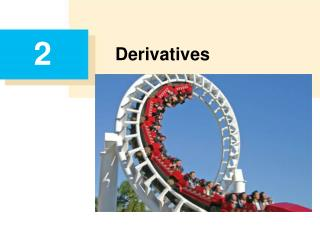DownloadDownload PresentationDerivatives

# Derivatives

Télécharger la présentation## Derivatives

- - - - - - - - - - - - - - - - - - - - - - - - - - - E N D - - - - - - - - - - - - - - - - - - - - - - - - - - -
##### Presentation Transcript

1. 2 Derivatives

2. 2.1 Derivatives and Rates of Change

3. Tangents

4. Tangents

5. Rates of Change and Tangent

6. Example 1 • Find an equation of the tangent line to the parabola y = x2 at the point P(1, 1). • Solution: • Here we have a = 1 and f(x) = x2, so the slope is

7. Example 1 – Solution cont’d • Using the point-slope form of the equation of a line, we find that an equation of the tangent line at (1, 1) is • y – 1 = 2(x – 1) or y = 2x – 1

8. Tangents • We sometimes refer to the slope of the tangent line to a curve at a point as the slope of the curve at the point. • The idea is that if we zoom in far enough toward the point, the curve looks almost like a straight line. • Figure 2 illustrates this procedure for the curve y = x2 in Example 1. Zooming in toward the point (1, 1) on the parabola y = x2 Figure 2

9. Tangents • The more we zoom in, the more the parabola looks like a line. • In other words, the curve becomes almost indistinguishable from its tangent line. • There is another expression for the slope of a tangent line that is sometimes easier to use.

10. Velocities

11. Average Velocity: Velocity = Displacement / Time • which is the same as the slope of the secant line PQ:

12. Instantaneous Velocity: • Now suppose we compute the average velocities over shorter and shorter time intervals [a, a + h]. • In other words, we let h approach 0. As in the example of the falling ball, we define the velocity (or instantaneous velocity) v(a) at time t = a to be the limit of these average velocities: • This means that the velocity at time t = a is equal to the slope of the tangent line at P.

13. Example • Suppose that a ball is dropped from the upper observation deck of the CN Tower, 450 m above the ground. • (a) What is the velocity of the ball after 5 seconds? • (b) How fast is the ball traveling when it hits the ground? • Solution: • We will need to find the velocity both when t = 5 and when the ball hits the ground, so it’s efficient to start by finding the velocity at a general time t = a.

14. Example – Solution cont’d • Using the equation of motion s = f(t) = 4.9t2, we have

15. Example – Solution cont’d = 49 m/s. • (a) The velocity after 5 s is v(5) = (9.8)(5) • (b) Since the observation deck is 450 m above the ground, the ball will hit the ground at the time t1 when s(t1) = 450, that is, • 4.9t12 = 450 • This gives • t12 = and t1 =  9.6 s • The velocity of the ball as it hits the ground is therefore • v(t1) = 9.8t1 = 9.8  94 m/s

16. Derivative at x=a

17. Example • Find the derivative of the function f(x) = x2 – 8x + 9 at the number a. • Solution: • From the definition we have

18. Example – Solution cont’d

19. More general: Derivative at any x